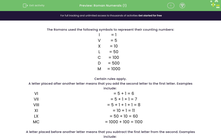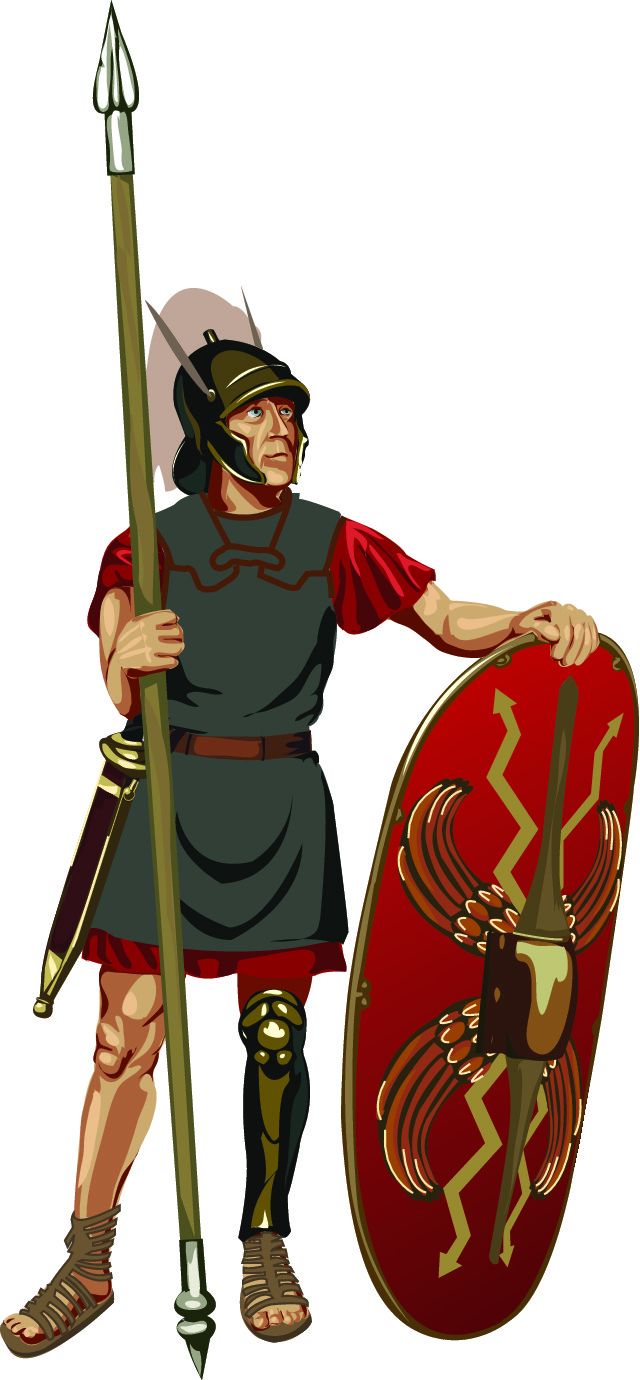# Interpret Roman Numerals

In this worksheet, students will convert from Roman numerals to normal numbers.Key stage:  KS 2

Curriculum topic:   Number: Number and Place Value

Curriculum subtopic:   Read Roman Numerals to M (1000)

Popular topics:   Numbers worksheets

Difficulty level:#### Worksheet Overview

The Romans used the following symbols to represent their counting numbers:

 I = 1 V = 5 X = 10 L = 50 C = 100 D = 500 M = 1000Certain rules apply.

A letter placed after another letter means that you add the second letter to the first letter. Examples include:

 VI = 5 + 1 = 6 VII = 5 + 1 + 1 = 7 VIII = 5 + 1 + 1 + 1 = 8 XI = 10 + 1 = 11 LX = 50 + 10 = 60 MC = 1000 + 100 = 1100

A letter placed before another letter means that you subtract the first letter from the second. Examples include:

 IV = 5 - 1 = 4 IX = 10 - 1 = 9 XL = 50 - 10 = 40 CM = 1000 - 100 = 900

Example

For what number does this Roman numeral stand?

MMCMXLIX

MM = 2000

CM = 1000 - 100 = 900

XL = 50 - 10 = 40

IX = 10 - 1 = 9

So MMCMXLIX = 2949

It may look complicated, but just take it one step at a time and you'll soon get the hang of it.

You can look back at this introduction at any point by clicking on the red help button on the screen.Let's get  started.

### What is EdPlace?

We're your National Curriculum aligned online education content provider helping each child succeed in English, maths and science from year 1 to GCSE. With an EdPlace account you’ll be able to track and measure progress, helping each child achieve their best. We build confidence and attainment by personalising each child’s learning at a level that suits them.

Get started